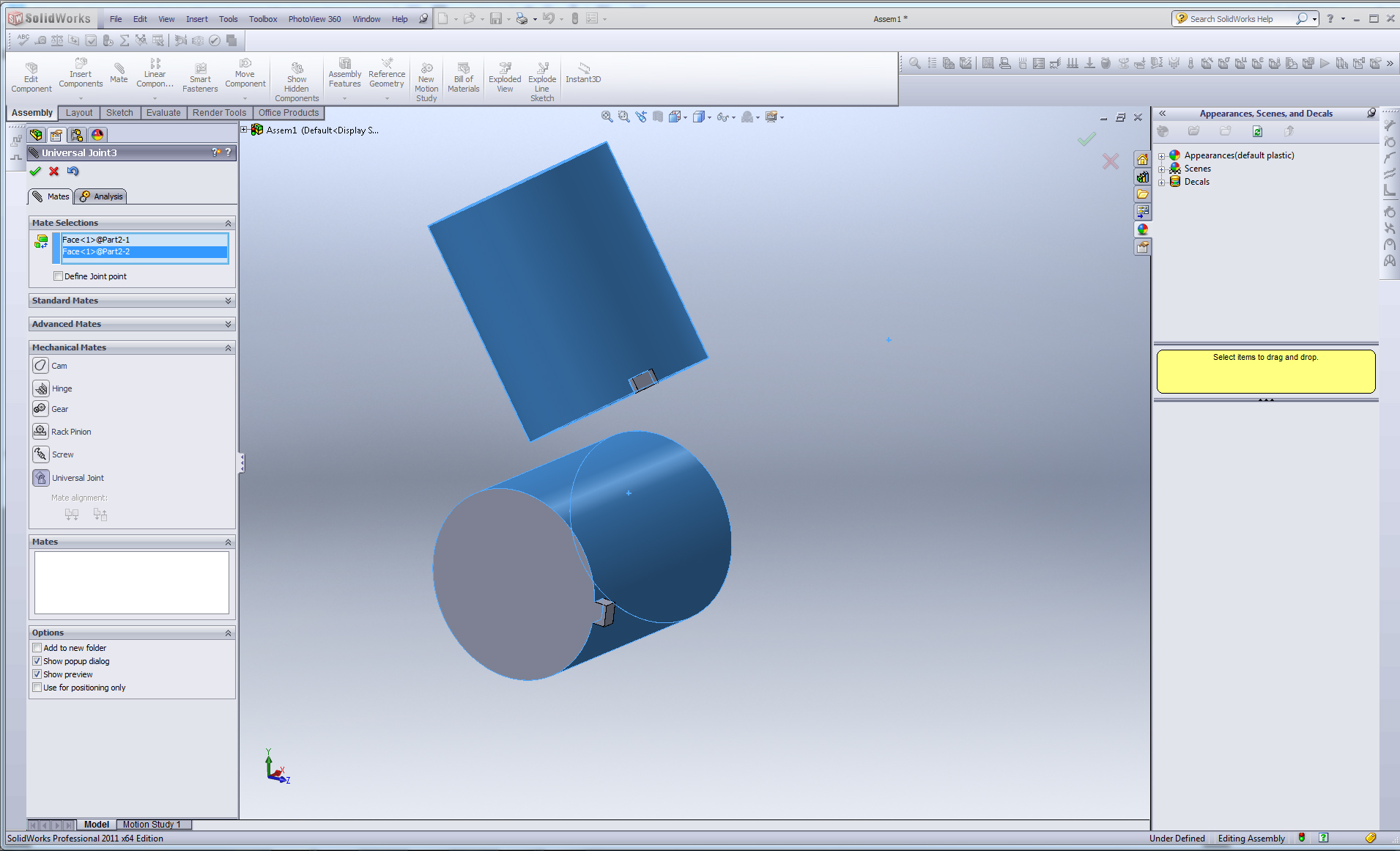# Is it possible to mate circular faces?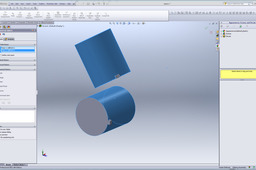You can use several types of mates in SolidWorks to do this other than simple concentric, depending on what effect you want. Here are some examples, although I'm sure I've left some out :)

1. ### Step 1:

Tangent faces will bring the two faces so that they touch.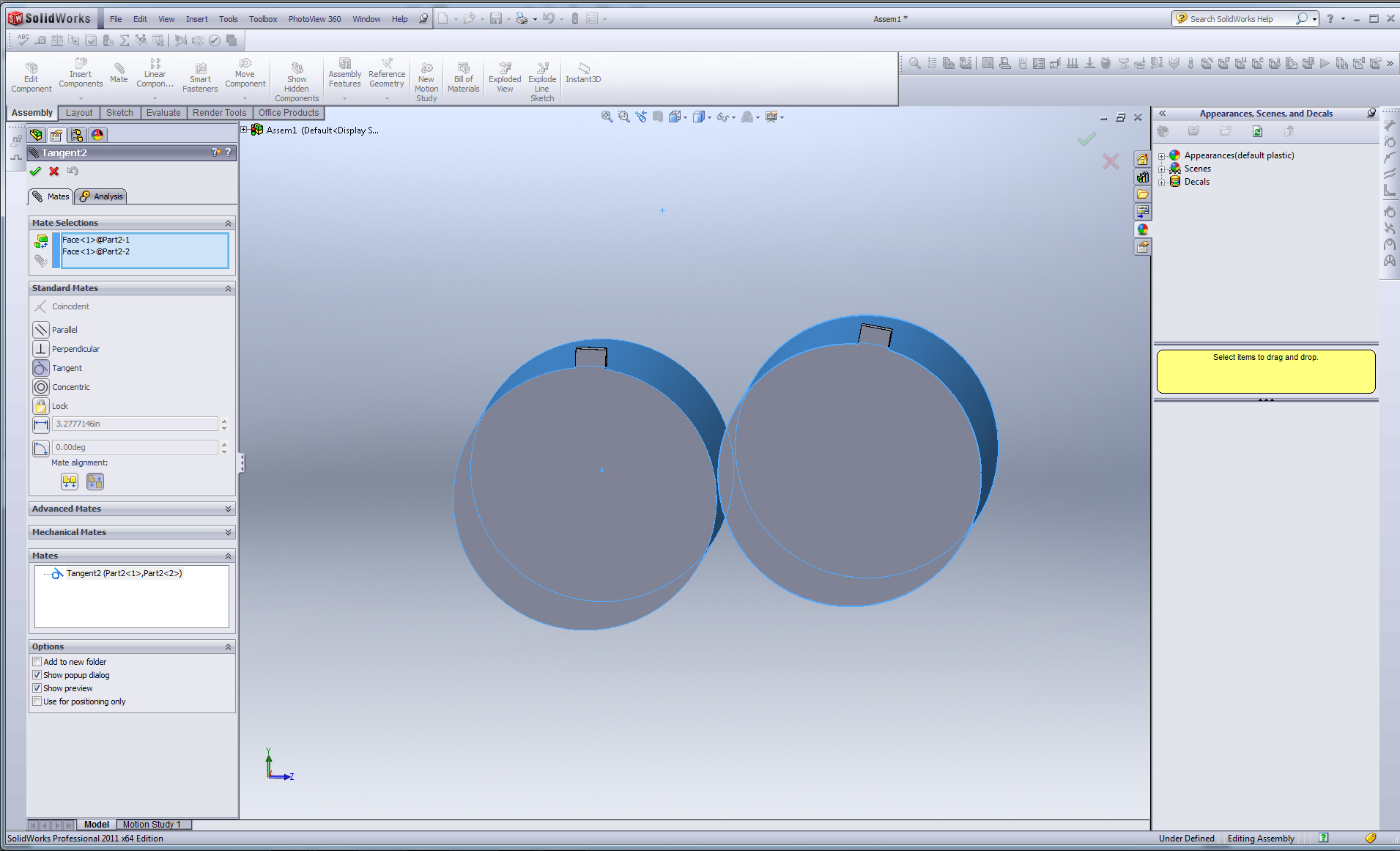2. ### Step 2:

With a tangent mate, the two parts are free to be rotated around one another. Other mates can be used to keep them fixed at a certain position (parallel or coincident planes, end faces, axes, etc.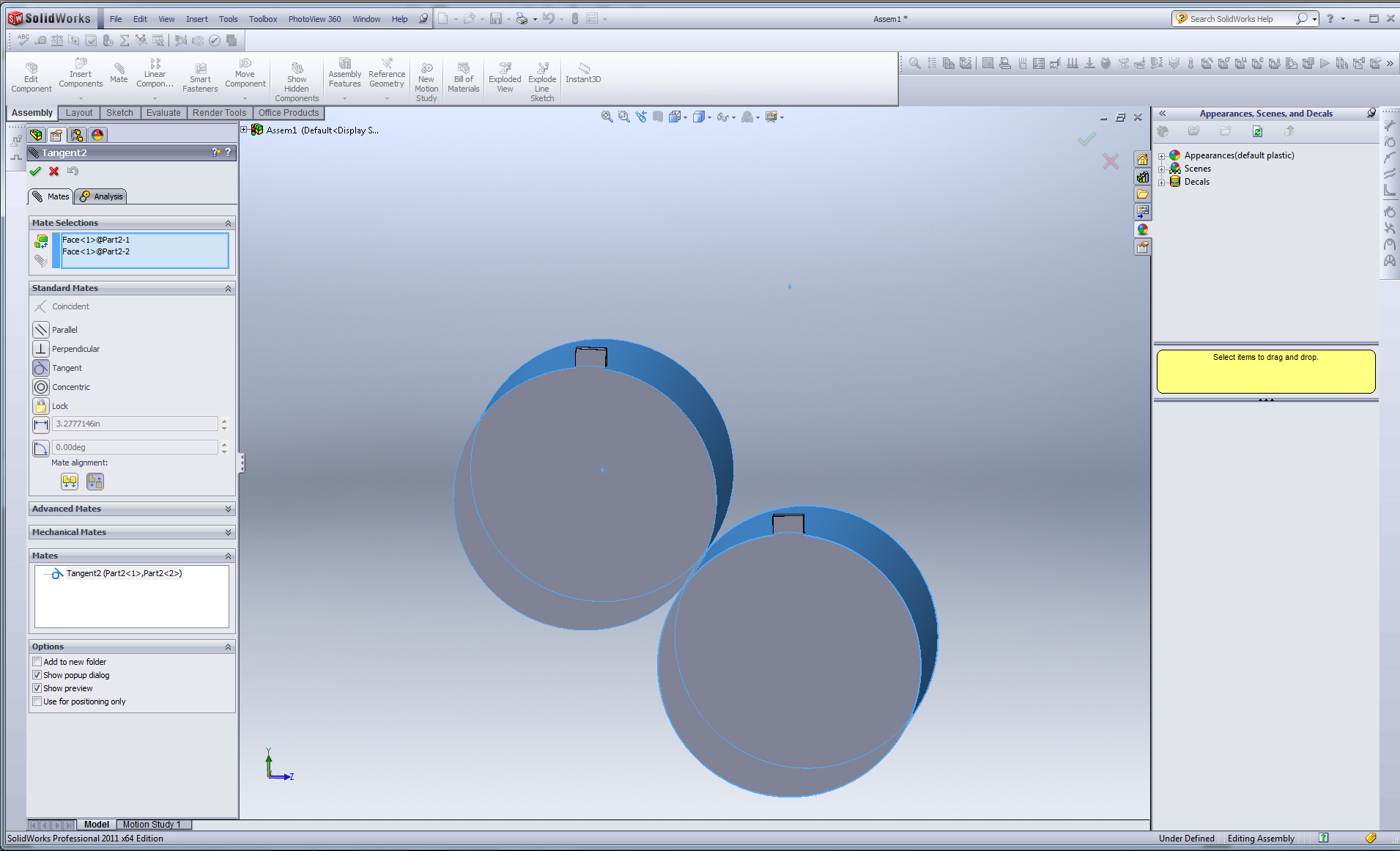3. ### Step 3:

Gear Mates (Under Mechanical Mates) can be used so that the two faces rotate relative to one another.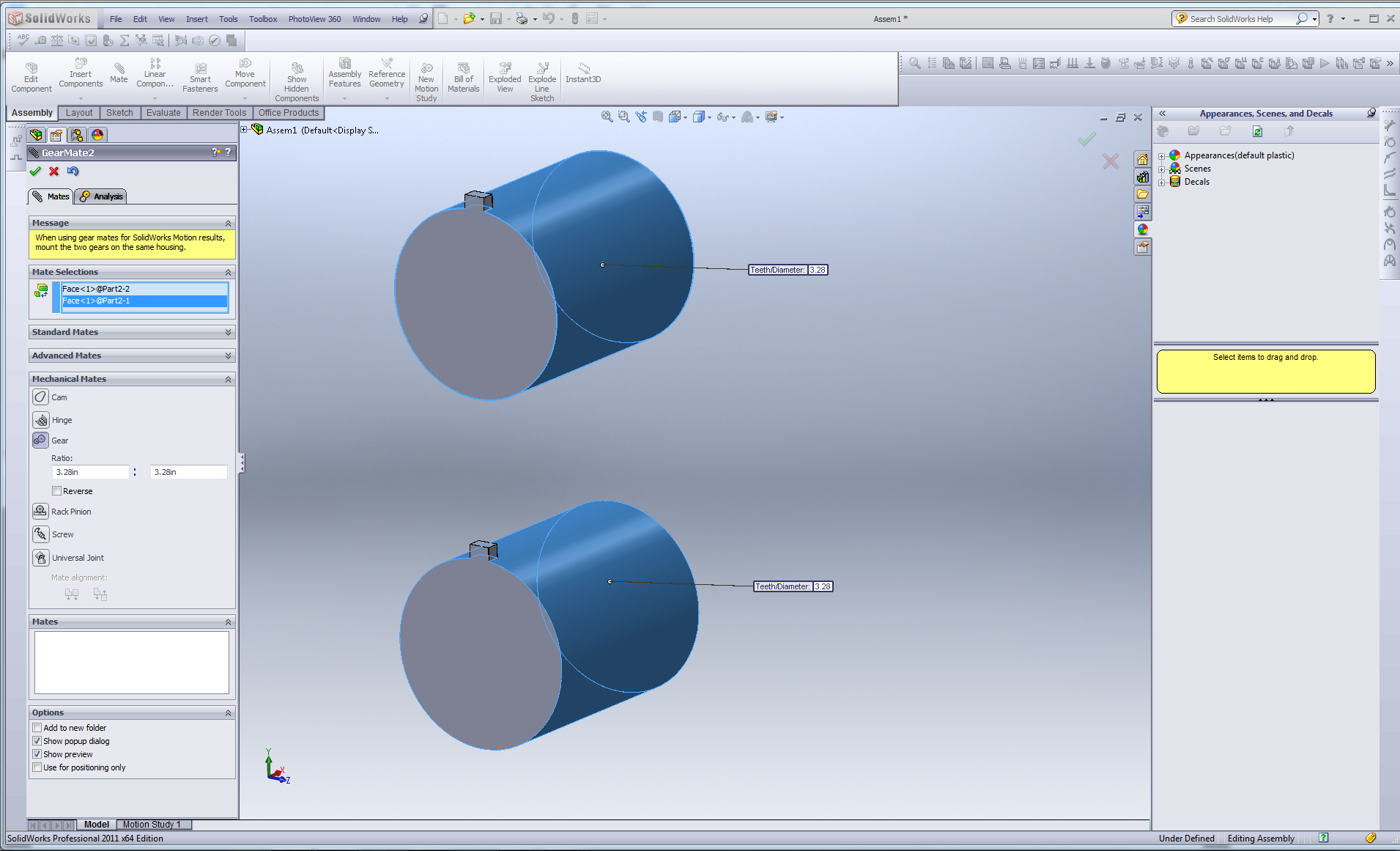4. ### Step 4:

As shown here, the parts rotate oppositely when one or the other is rotated, as if they were connected by gear teeth.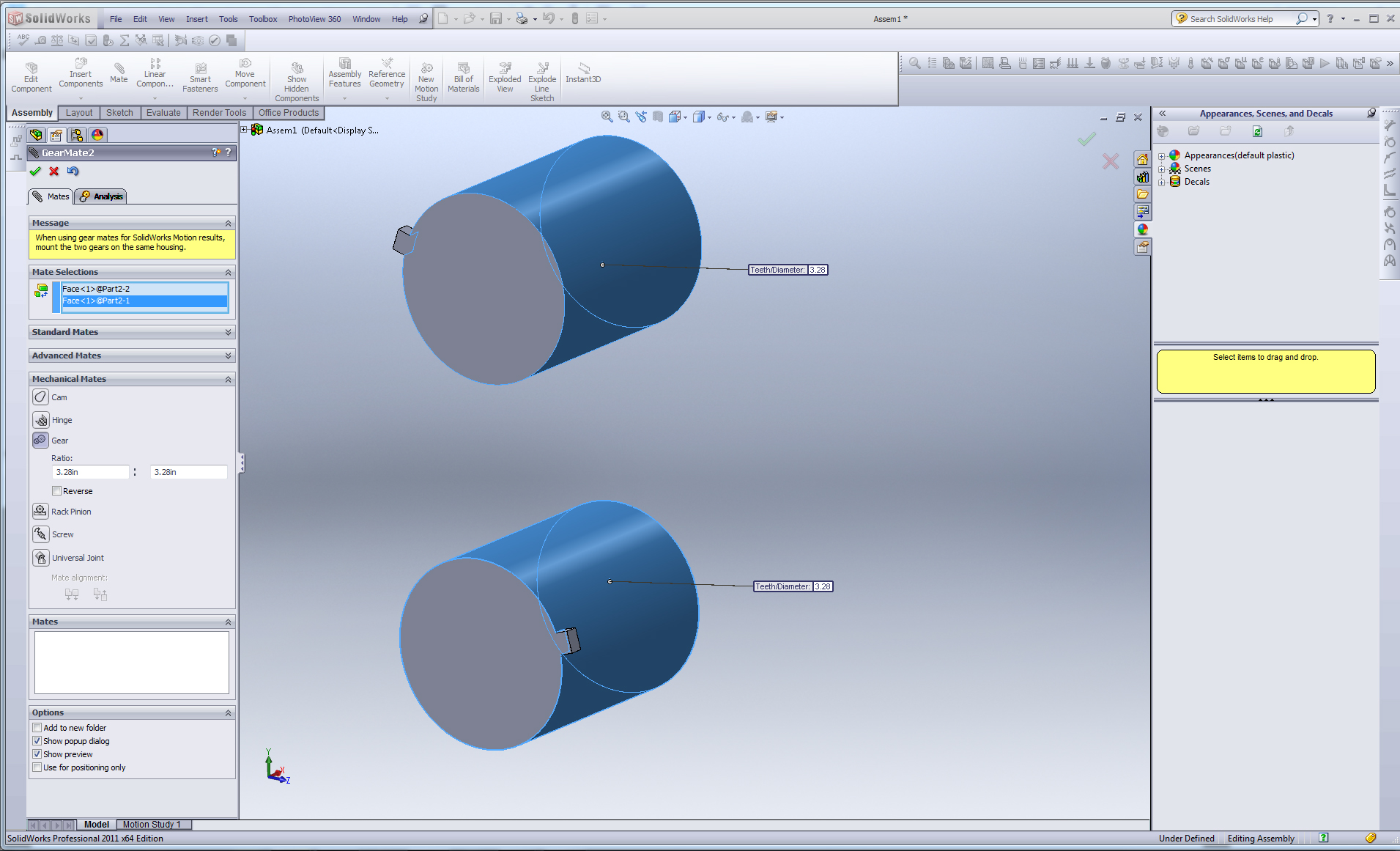5. ### Step 5:

Gear ratios can be set so that the rotations are set at a certain ratio to one another.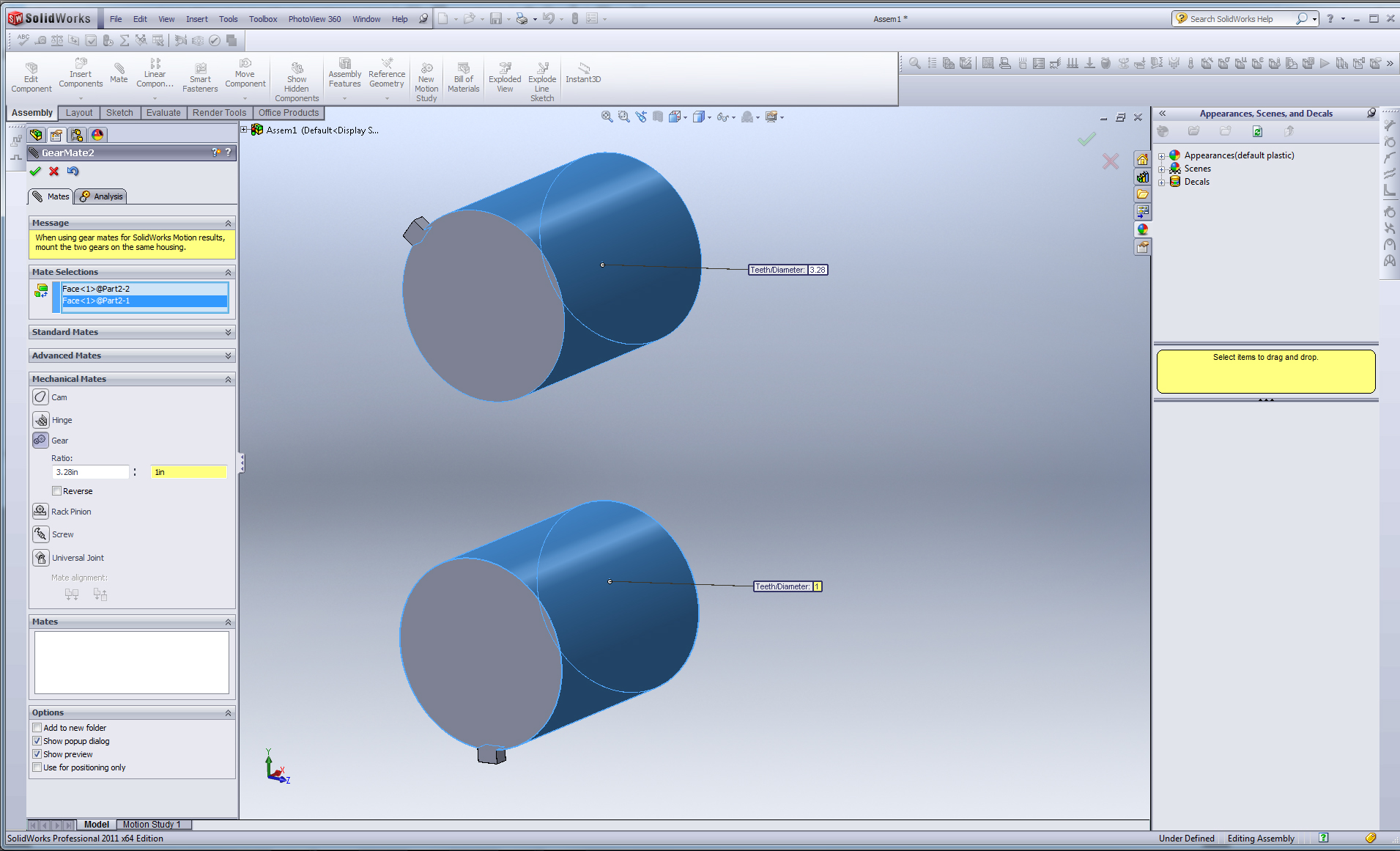6. ### Step 6:

Screw mates can be used if the two faces are desired to be concentric.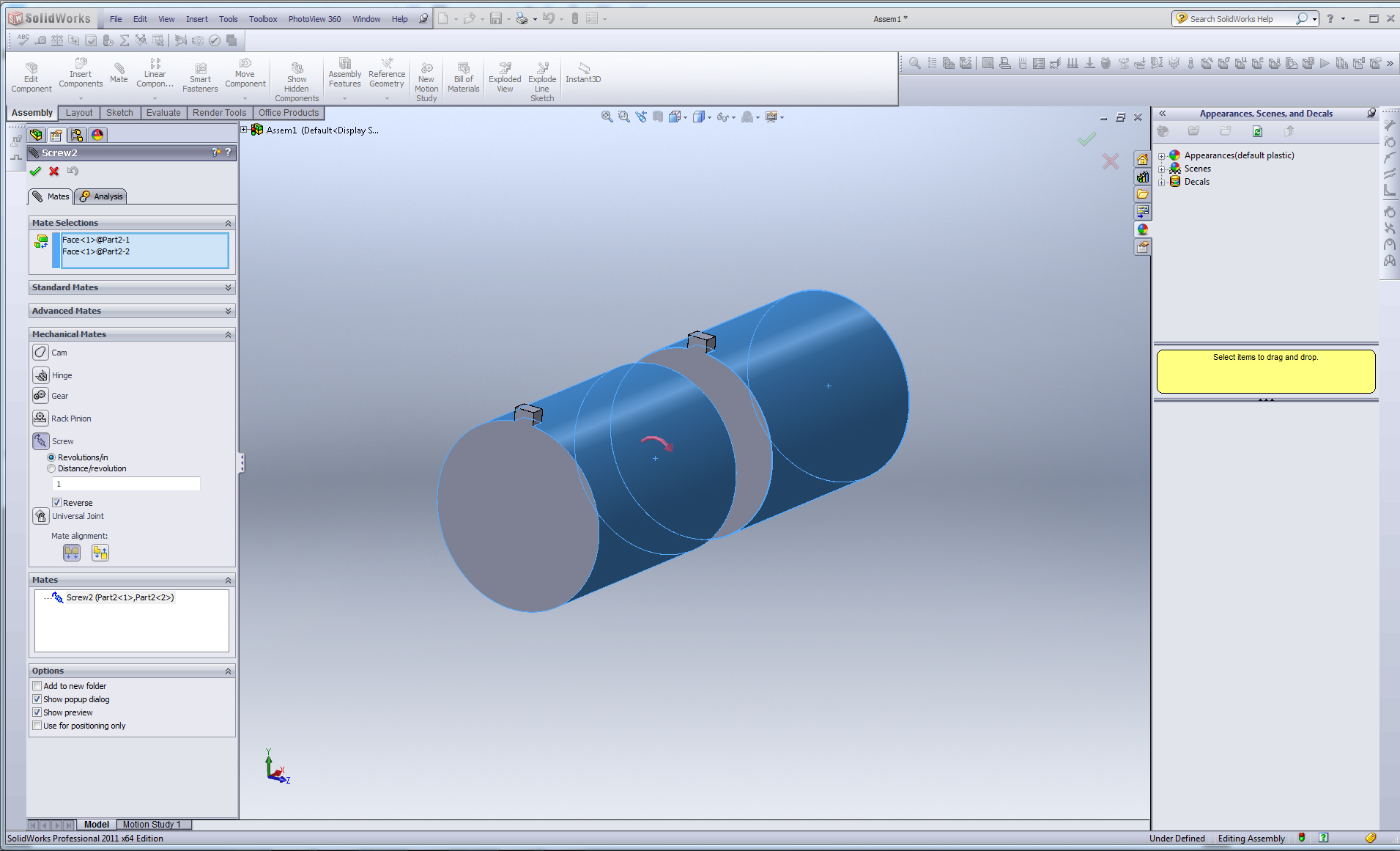7. ### Step 7:

With screw mates, the two parts will rotate together.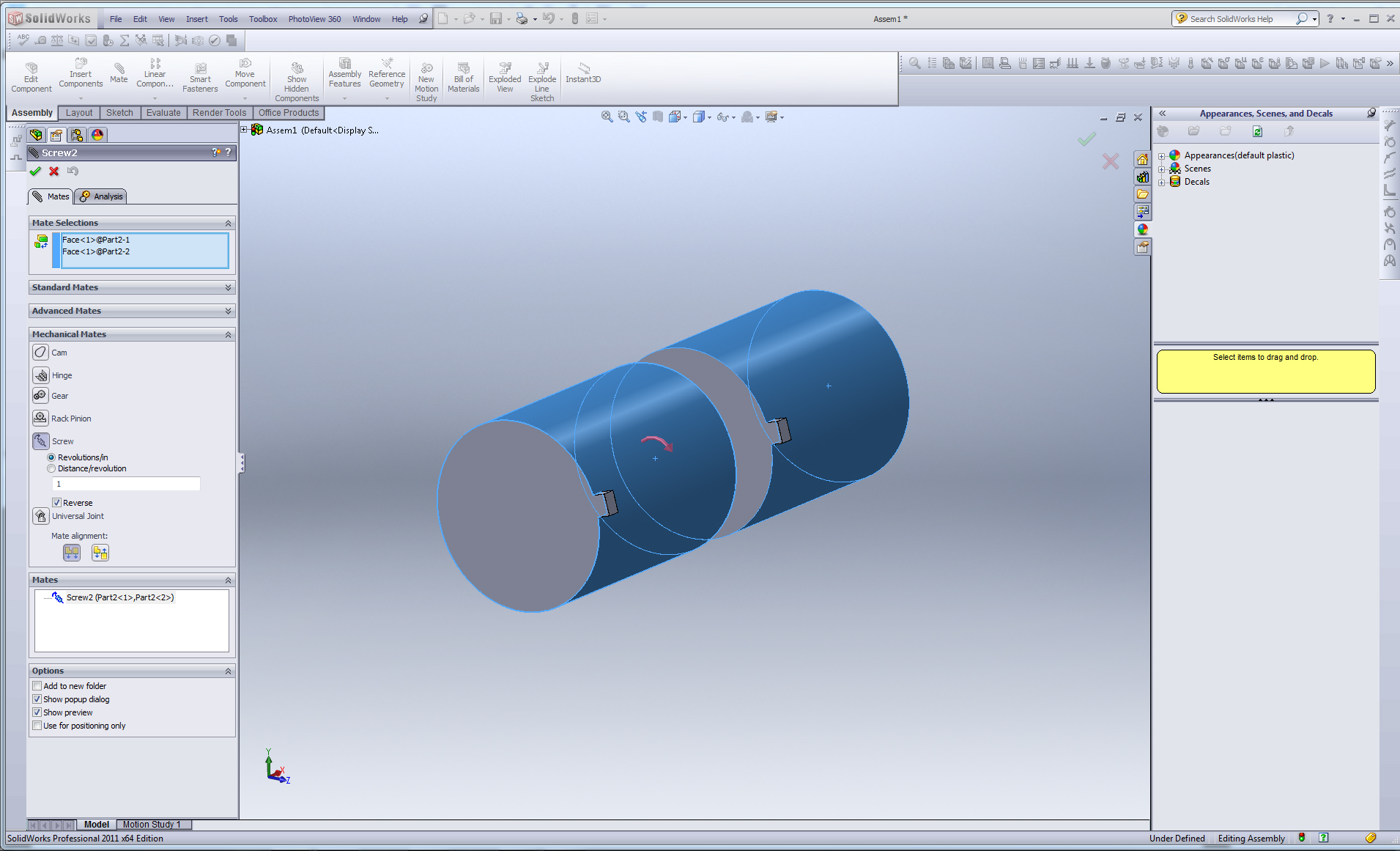8. ### Step 8:

Universal Joint mates are used when two circular faces are neither tangent nor concentric.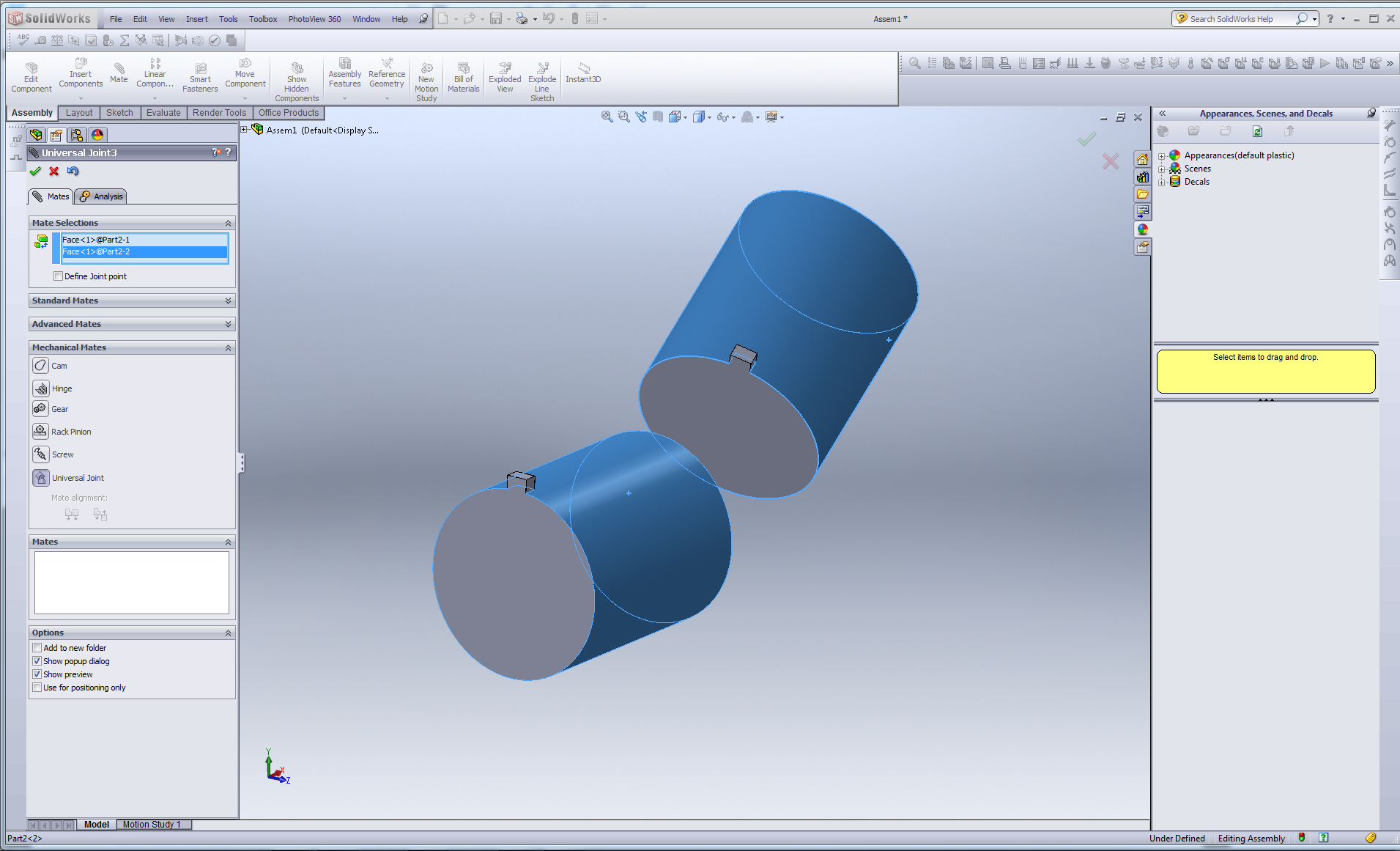9. ### Step 9:

The parts will rotate relative to one another as if connected by a universal joint.